# 978-0123869449 Chapter 8 Part 2

Document Type
Homework Help
Book Title
Authors
Michael F. Modest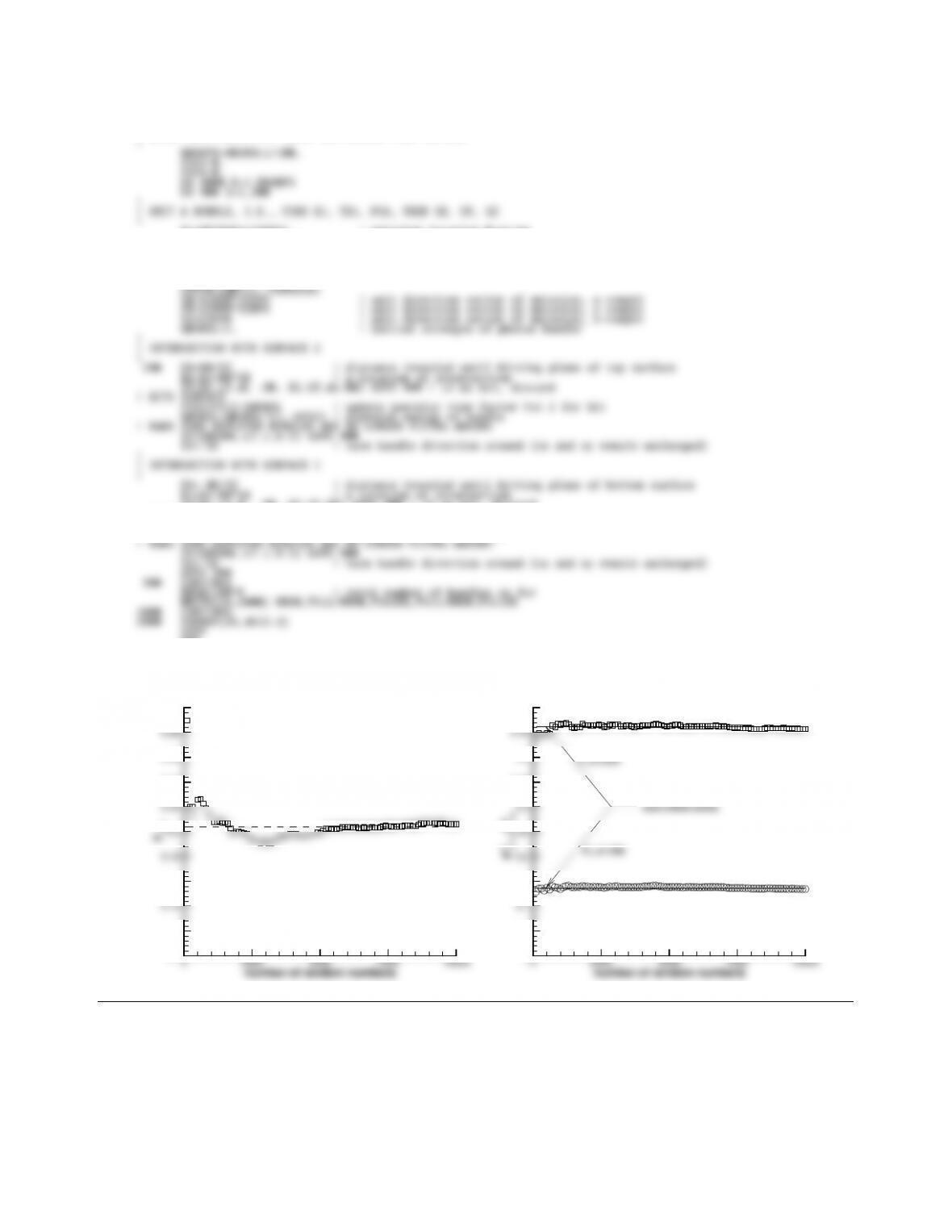CHAPTER 8 253
WRITE(LU,*) ’ZONE’
!
! BREAK INTO GROUPS OF 100S, INITIALIZE VIEW FACTORS
PSI=2.*PI*RAN1(ISEED) ! azimuthal angle of emission
COSPS=COS(PSI)
SINPS=SIN(PSI)
SINSQTH=RAN1(ISEED) ! sinˆ2 of polar angle of emission
SINTH=SQRT(SINSQTH)
IF(X1.LT.0. .OR. X1.GT.WW) GOTO 900 ! if no hit, discard
! HITS SURFACE
FS11=FS11+QBUNDL ! update specular view factor Fs1-1 for hit
QBUNDL=QBUNDL*(1.-EPS1) ! diminish energy of bundle
END
F1-2
0.1
0.12
0.16
0.24
0.26
0.3
1-2
0
0.05
0.15
0.35
0.4
0.5
1-1=0.1366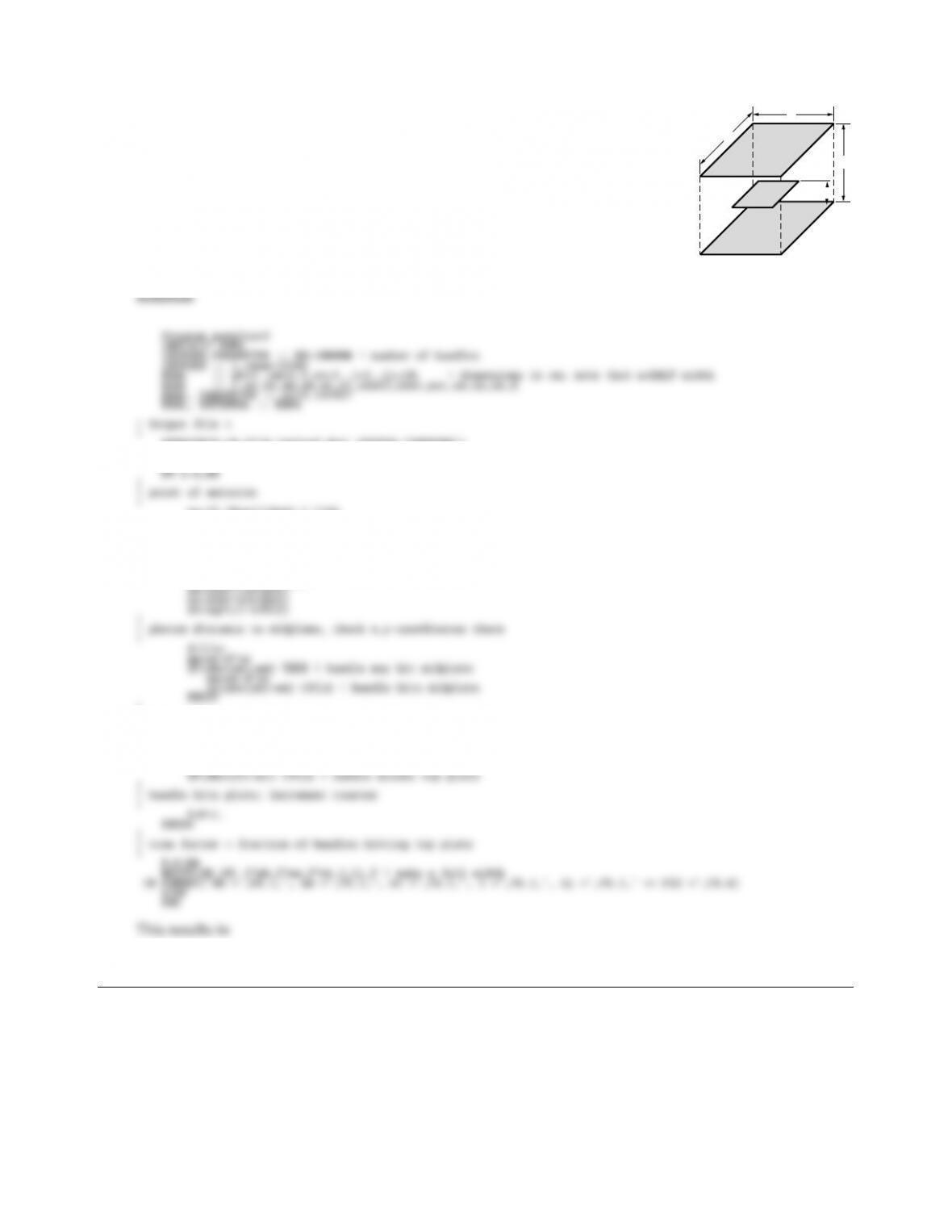8.10
w
w
L
A2
A1
l
b
b
Two directly opposed quadratic plates of width w=10cm are spaced a distance
L=10 cm apart, with a third centered quadratic plate of dimension b×b(b=
5 cm) in between at a distance l=5 cm from the bottom. Determine the view
factor F12via Monte Carlo. In order to verify your code (and to have a more
ﬂexible tool) it may be best to allow for arbitrary and dierent top and bottom
was well as b.
F=0.
ye=(2.*Ran1(idum)-1.)*wb
!
! direction of emission
!sint2=Ran1(idum)
sint=sqrt(sint2)
psi=2*pi*Ran1(idum)
!
! photon distance to top plane, check x,y-coordinates there
!d=LL/sz
xt=xe+d*sx
IF(abs(xt)>wt) CYCLE ! bundle misses top plate
yt=ye+d*sy
wb = 10.0, wm = 5.0, wt = 10.0, l = 5.0, LL = 10.0 => F12 = 0.1012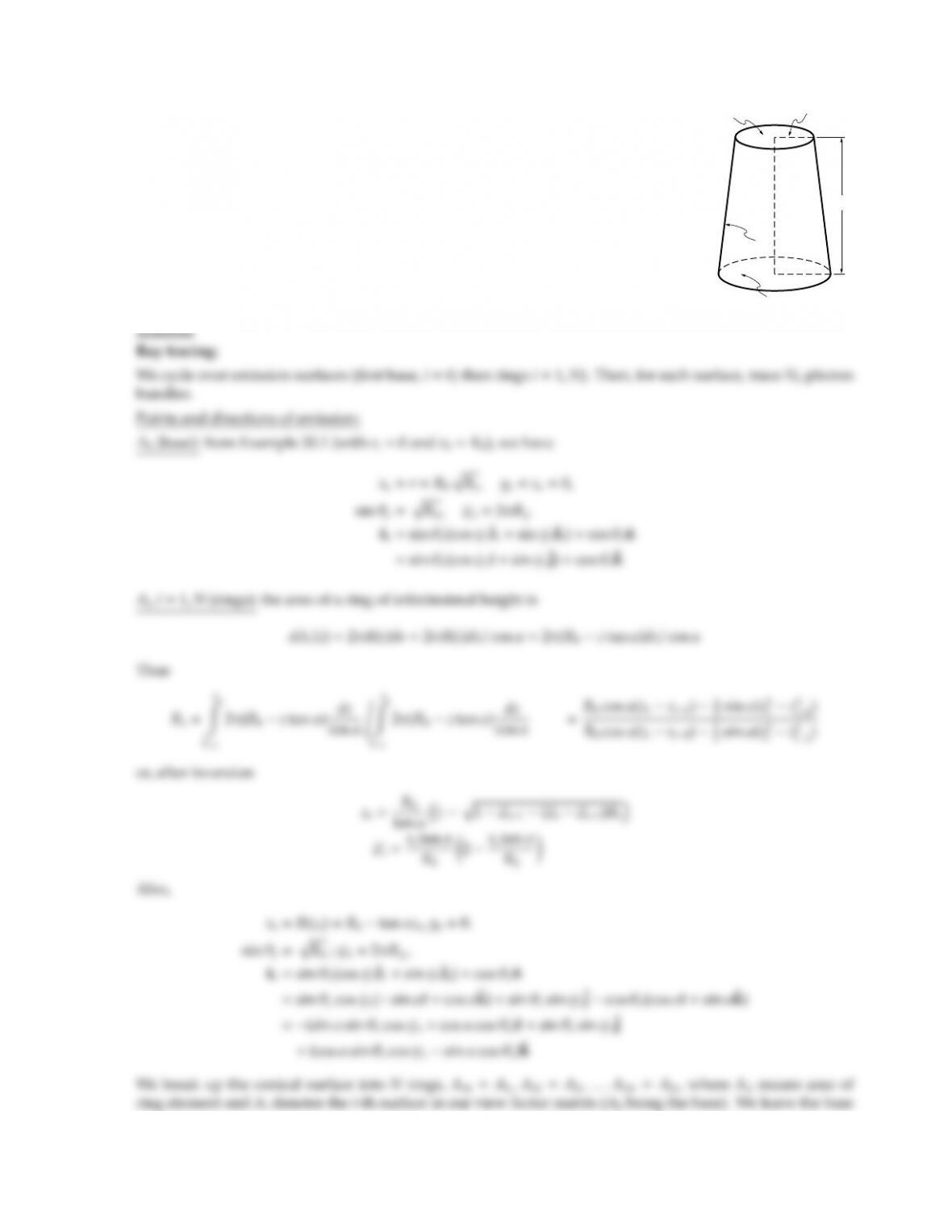CHAPTER 8 255
8.14
5 cm
2 cm
4 cm
A2
A3
A1
Consider the conical geometry of Problem 5.9: breaking up the sidewall into strips
(say 4), calculate all relevant view factors (base-to-rings, ring-to-rings) via Monte
Carlo.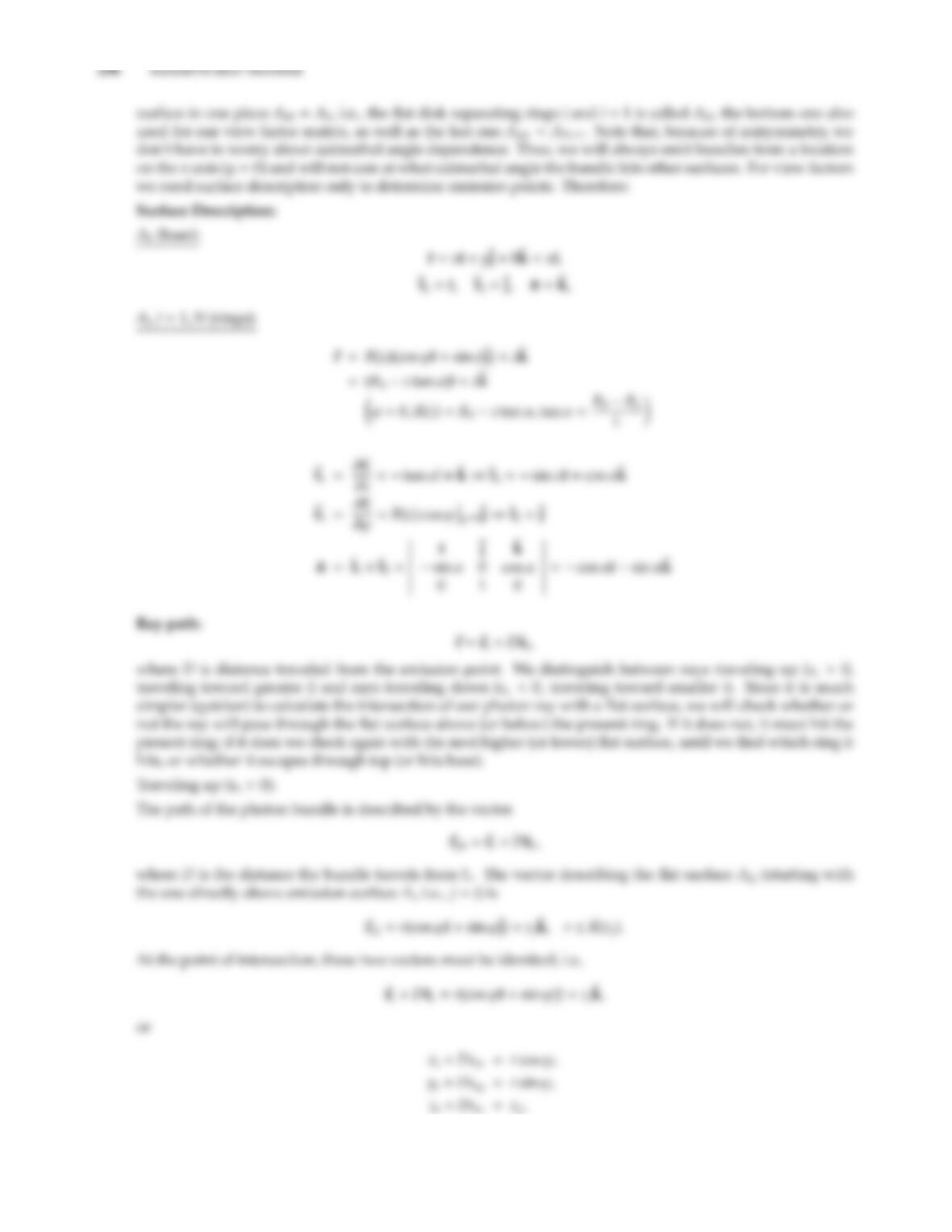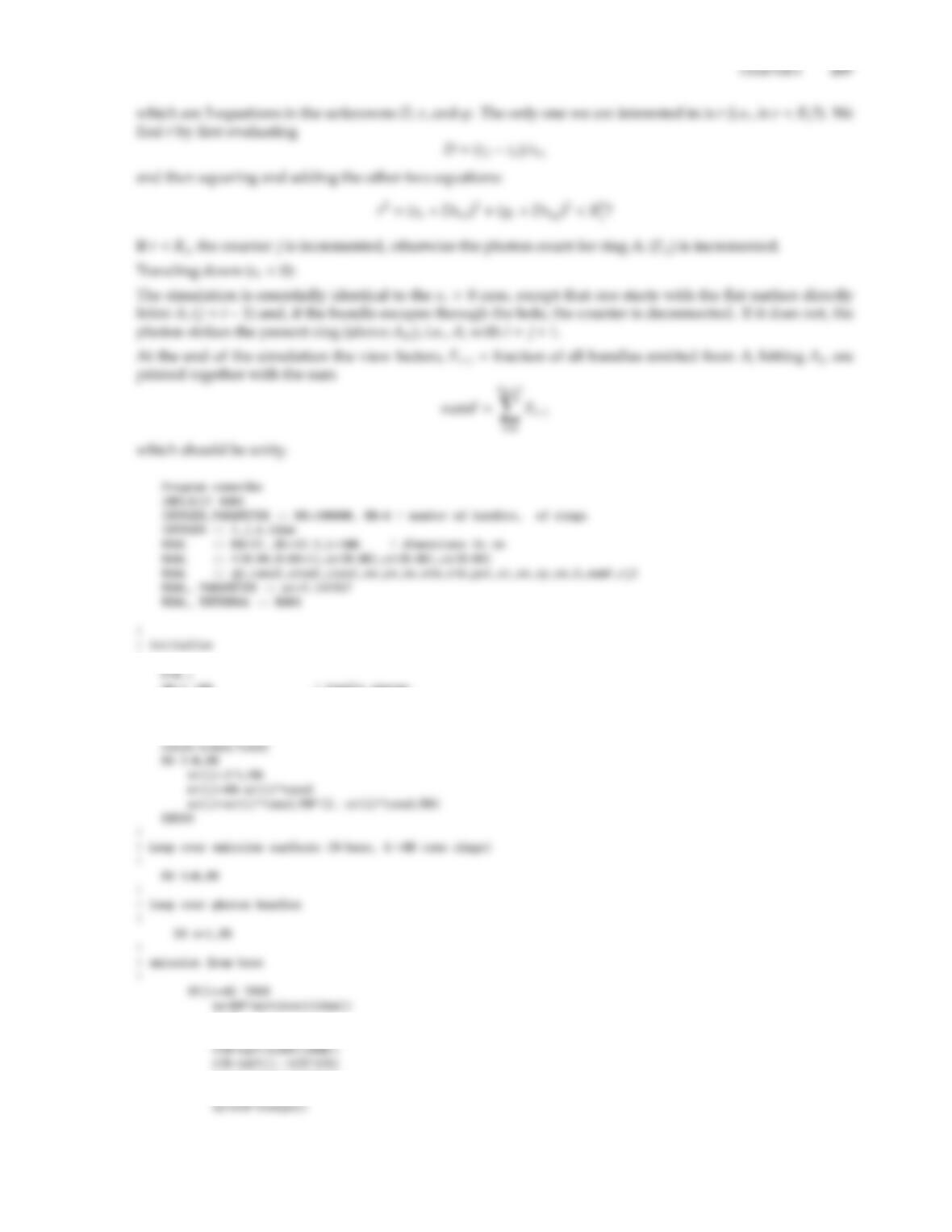!
qb=1./NB ! bundle energy
! set ring heights and radii
tanal=(R0-RL)/L ! tangent of angle: can approach but not reach 0 to model cylinder
sinal=(R0-RL)/sqrt((R0-RL)**2+L**2)
ye=0.
ze=0.
psi=2.*pi*ran1(idum)
sx=sth*cos(psi)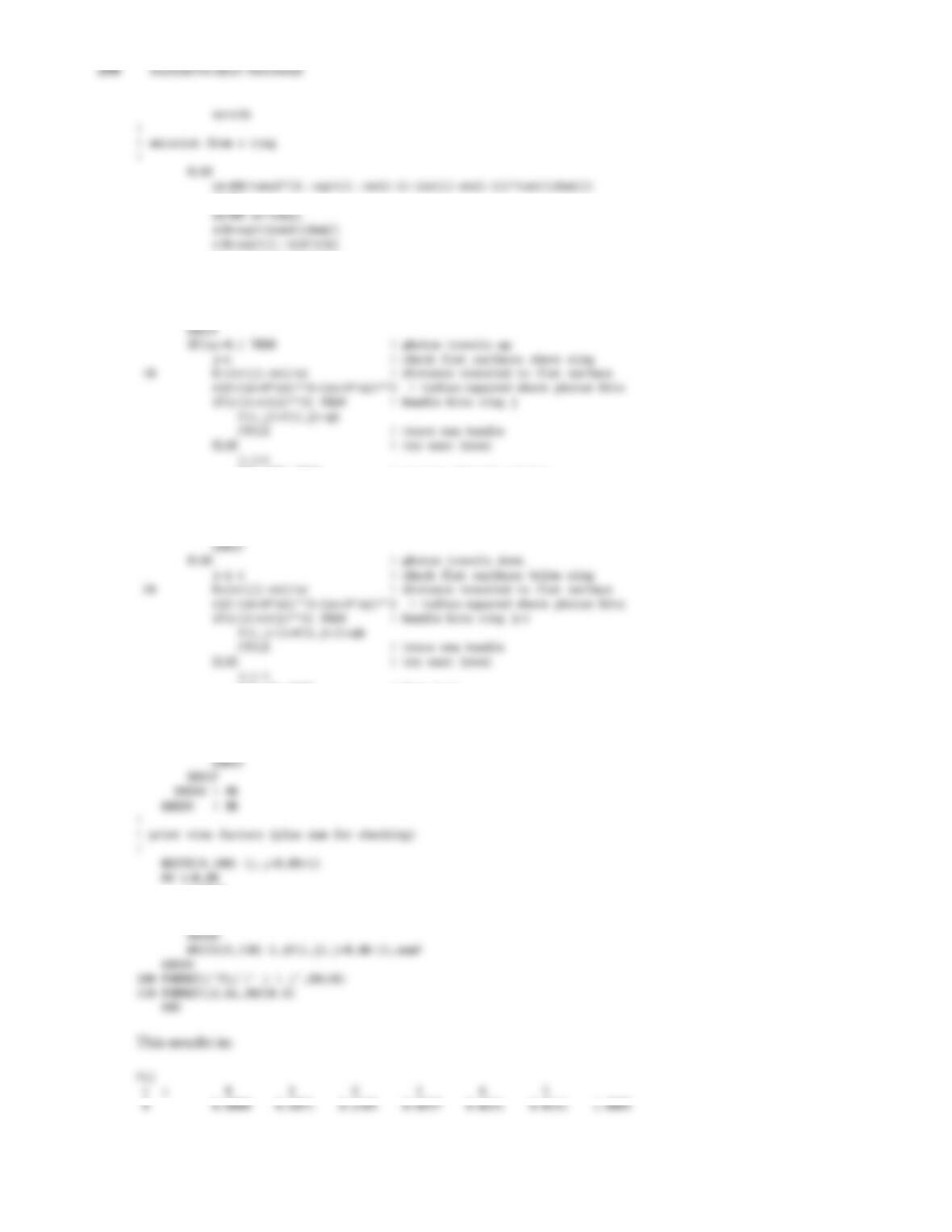ye=0.
psi=2.*pi*ran1(idum)
s1=sth*cos(psi)
sy=sth*sin(psi)
sx=-sinal*s1-cosal*cth
sz= cosal*s1-sinal*cth
IF(j>NR) THEN ! escapes through opening
F(i,NR+1)=F(i,NR+1)+qb
CYCLE ! trace new bundle
ENDIF
GOTO 10
IF(j<0) THEN ! hits base
F(i,0)=F(i,0)+qb
CYCLE ! trace new bundle
ENDIF
GOTO 20
sumF=0.
DO j=0,NR+1
sumF=sumF+F(i,j)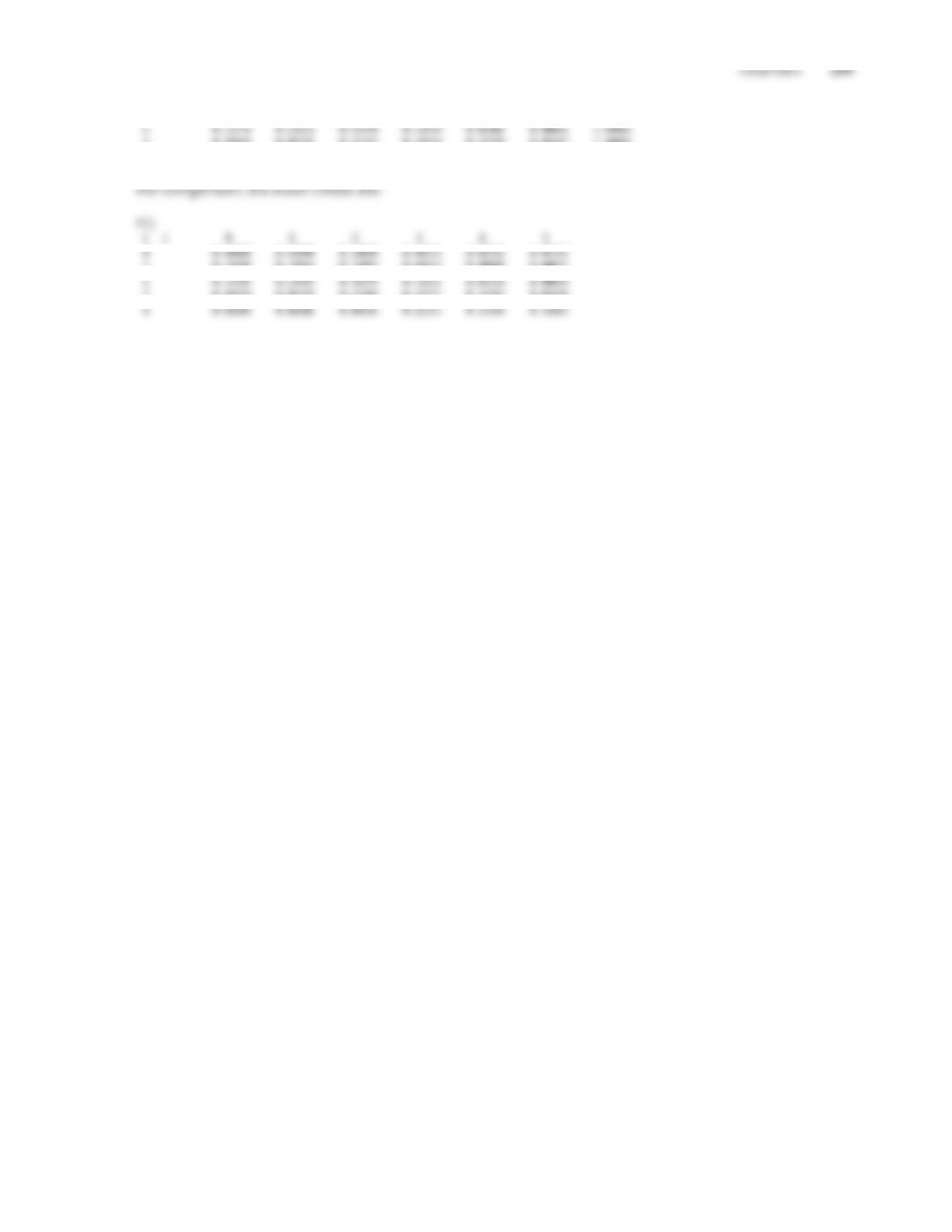1 0.3643 0.3960 0.1870 0.0415 0.0088 0.0026 1.0003
3 0.0469 0.0572 0.2122 0.4854 0.1738 0.0247 1.0002
4 0.0207 0.0144 0.0441 0.2094 0.5435 0.1684 1.0003
1 0.3698 0.3864 0.1903 0.0423 0.0088 0.0025
3 0.0479 0.0576 0.2189 0.4771 0.1747 0.0238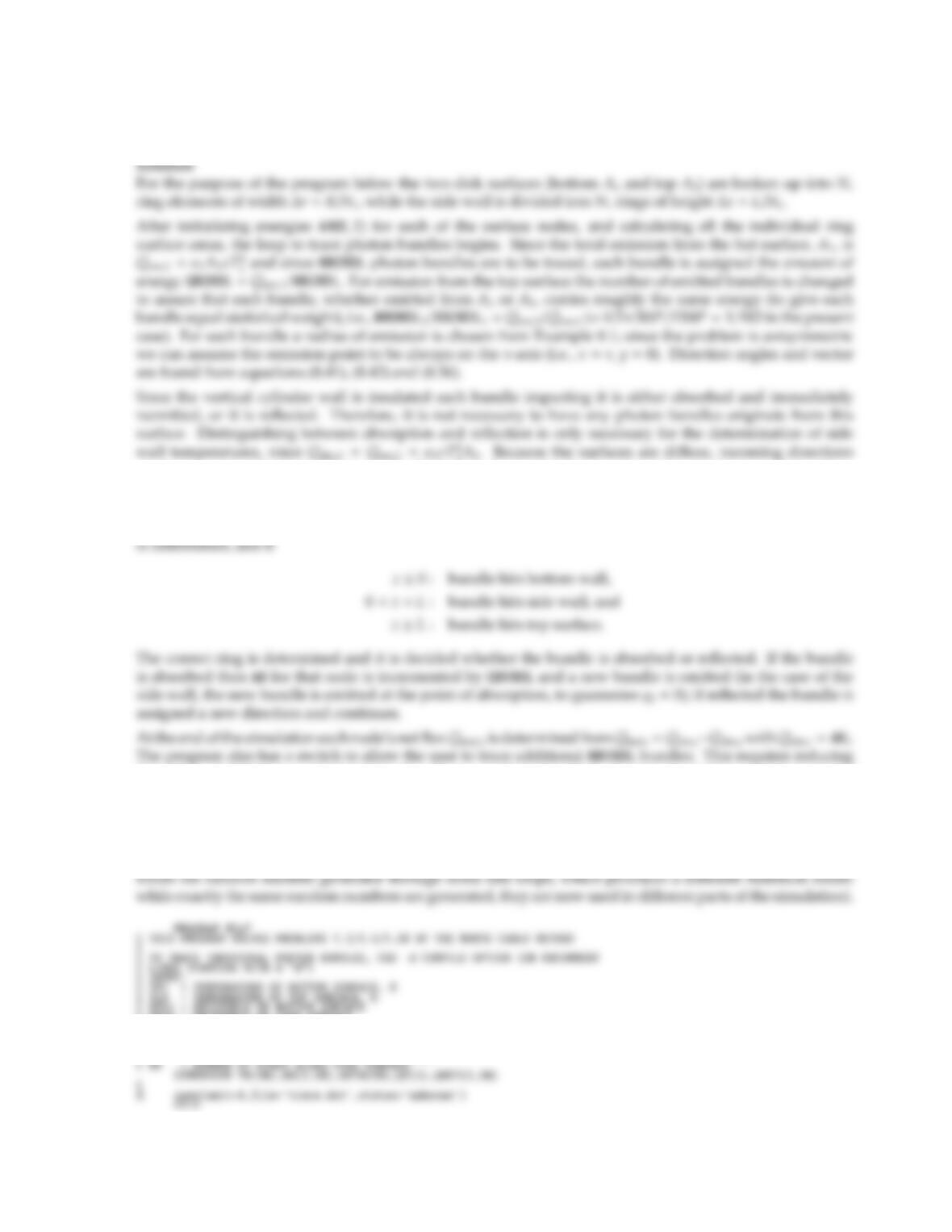8.15 Reconsider Problem 5.30:(a) find the solution by writing a small Monte Carlo program, and (b) augment this
program to allow for nongray, temperature-dependent emittances.
2A2. Because the surfaces are diuse, incoming directions
are irrelevant and finding directions of reﬂection is identical to finding directions of emission. For surface
A1ˆn1=ˆ
kand, therefore, sz=cos θ, for surface A3ˆn3=ˆ
kand sz=cos θ, while for the side wall A2ˆn2=ˆı
(negative x-direction) and sx=cos θ. In the actual code any bundle (whether leaving surface A1,A2or A3) is
traced to the point where it intersects the cylinder wall (assumed infinitely long). The z-location of that point
the values of QBUNDL accordingly (including the ones collected in AH during the simulation up to that point).
The program for this problem is written in Fortran77, and has a number of lines starting with a d, which
Fortran77 normally treats as commentary; with the proper compiler option these lines may be made part of
the code, giving detailed information of the path traced by individual bundles, if desired.
The code is given below, together with results for NBUNDL =100,000 and IDLE =0 (dierent values for IDLE
C EPS2 = EMITTANCE OF SIDE SURFACE
C EPS1 = EMITTANCE OF TOP SURFACE
C RC = CYLINDER RADIUS, CM
C CL = CYLINDER LENGTH, CM
C NR = NUMBER OF RINGS ON TOP/BOTTOM SURFACES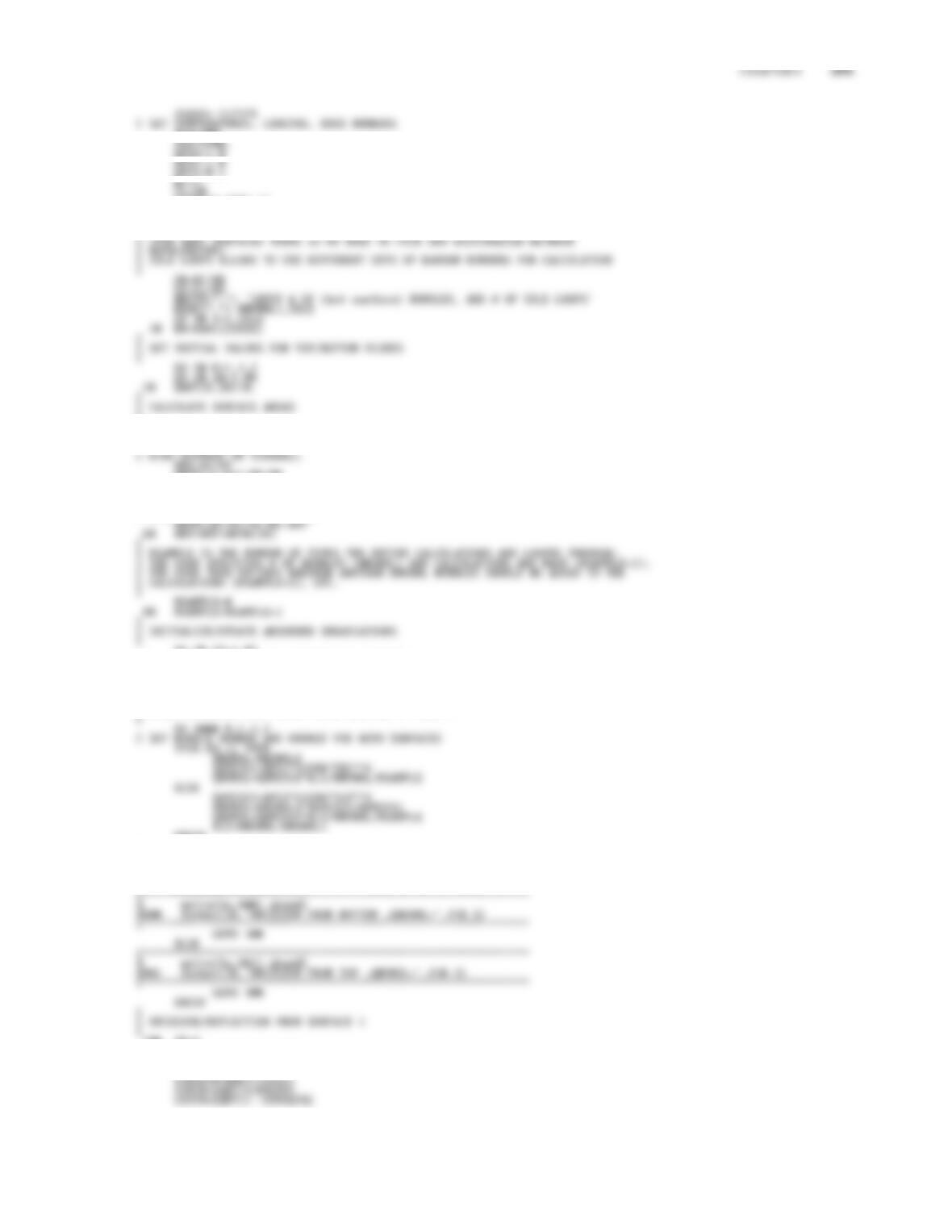TLO=500.
EPS1=1.0
EPS3=0.5
CL=20.
SIGMA=5.670E-12
NR=5
NZ=10
C
CPI=3.1415927
A13=PI*RC*RC
A2=2.*PI*RC*CL
ARTB(1)=A13/NR/NR
ART=ARTB(1)
DO 60 IR=2,NR
RL=RC*IR/NR
C RING ELEMENT ON TOP/BOTTOM
70 AH(2,IZ)=AH(2,IZ)*(NSAMPLE-1.)/NSAMPLE
DO 80 K=1,3,2
DO 80 IR=1,NR
80 AH(K,IR)=AH(K,IR)*(NSAMPLE-1.)/NSAMPLE
C
C START EMISSION LOOP FIRST OVER SURFACE 1, THEN 3
ENDIF
CDO 1000 IBUNDL=1,NBUNDL
RL=RC*SQRT(RAN1(ISEED))
IF(K.EQ.1) THEN
PSI=2.*PI*RAN1(ISEED)
COSPS=COS(PSI)
SINPS=SIN(PSI)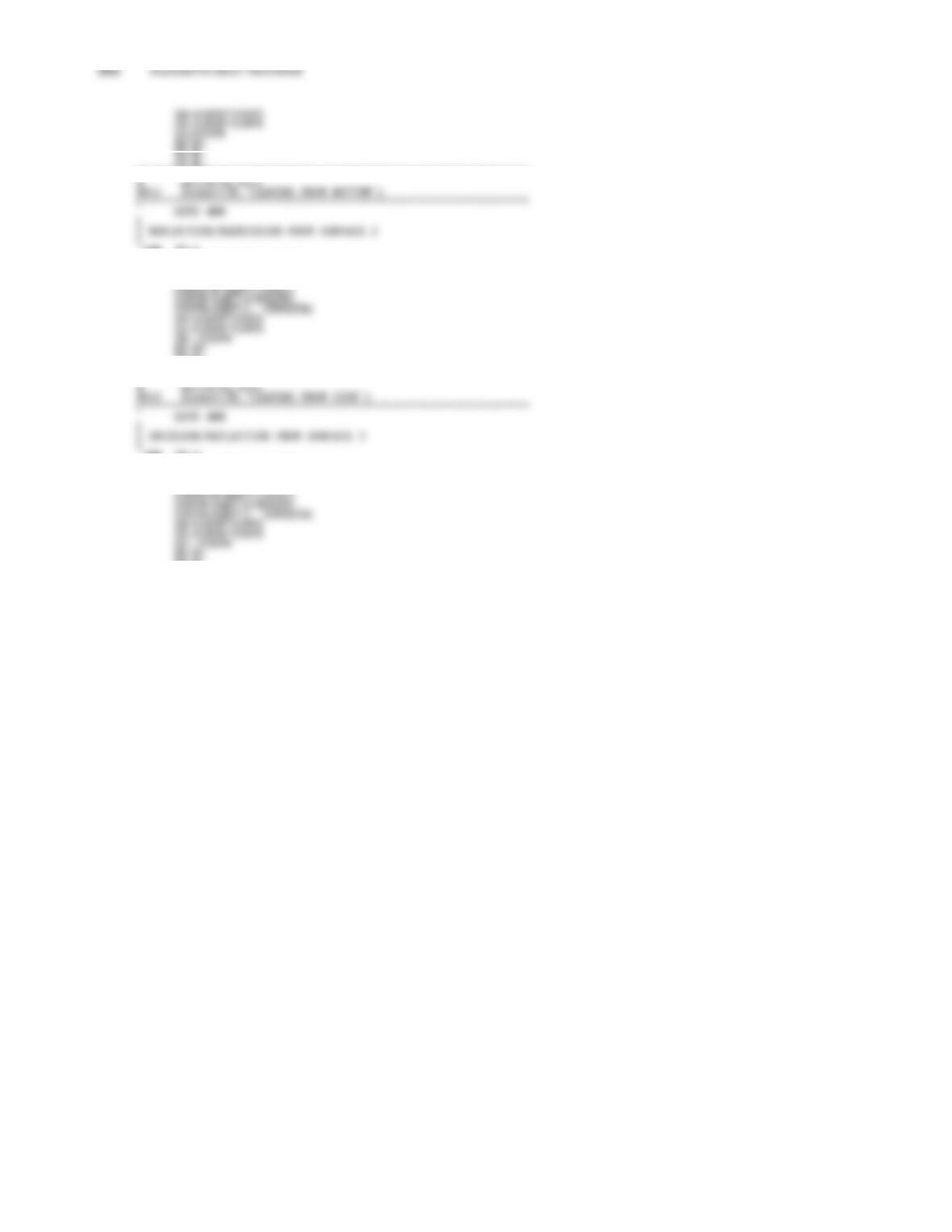ZE=0.
C*************************************************************
PSI=2.*PI*RAN1(ISEED)
COSPS=COS(PSI)
SINPS=SIN(PSI)
YE=0.
ZE=ZL
C*************************************************************
PSI=2.*PI*RAN1(ISEED)
COSPS=COS(PSI)
SINPS=SIN(PSI)
YE=0.
ZE=CL
C*************************************************************
d write(lu,913)
d913 format(3X,’LEAVING FROM TOP’)
C*************************************************************
C
C CHECK FOR POINT OF INTERSECTION WITH CYLINDER 2
C400 SXY=SX*SX+SY*SY
C****************************************************************
d th=180./pi*atan(sinth/costh)
d psid=180./pi*psi
d write(lu,904) ibundl,xe,ze,th,psid,sx,sy,sz
d 904 format(6X,’B#=’,I3,’ XE=’,F4.1,’ ZE=’,F5.1,
d 1 ’ TH=’,F4.0,’ PSI=’,F4.0,’ S=(’,3f6.3,’)’)
C****************************************************************
D=(SQRT(SXY*RC*RC-(XE*SY)**2)-XE*SX)/SXY
ZL=ZE+D*SZ
IF(ZL.LT.0.) THEN
GOTO 500
ELSEIF(ZL.GT.CL) THEN
GOTO 600
ELSE IZ=ZL/DZ+1
C*******************************************************************
d write(lu,906)zl,iz,d
d906 format(6X,’BUNDLE HITS SIDEWALL’,’ ZL=’,F5.1,’ IZ=’,I2,
d 1 ’ D=’,F4.1)
C*******************************************************************
C IS RAY ABSORBED OR REFLECTED?
IF(RAN1(ISEED).LT.EPS2) THEN
C
C ABSORPTION/REEMISSION FROM CYLINDER WALL
CAH(2,IZ)=AH(2,IZ)+QBUNDL
ENDIF
GOTO 200
ENDIF
C
C CHECK FOR POINT OF INTERSECTION WITH SURFACE 1
C500 D=-ZE/SZ
RL=SQRT((XE+D*SX)**2+(D*SY)**2)
C*******************************************************************
d write(lu,908)rl,d
d908 format(6X,’BUNDLE HITS BOTTOM’,’ RL=’,F5.1,’ D=’,F4.1)
C*******************************************************************
C IS RAY ABSORBED OR REFLECTED?
IF(RAN1(ISEED).LT.EPS1) THEN
C RAY IS ABSORBED
IR=RL/DR+1
AH(1,IR)=AH(1,IR)+QBUNDL
C*******************************************************************
d write(lu,921)ir
d921 format(6X,’BUNDLE IS ABSORBED’,’ IR=’,I2)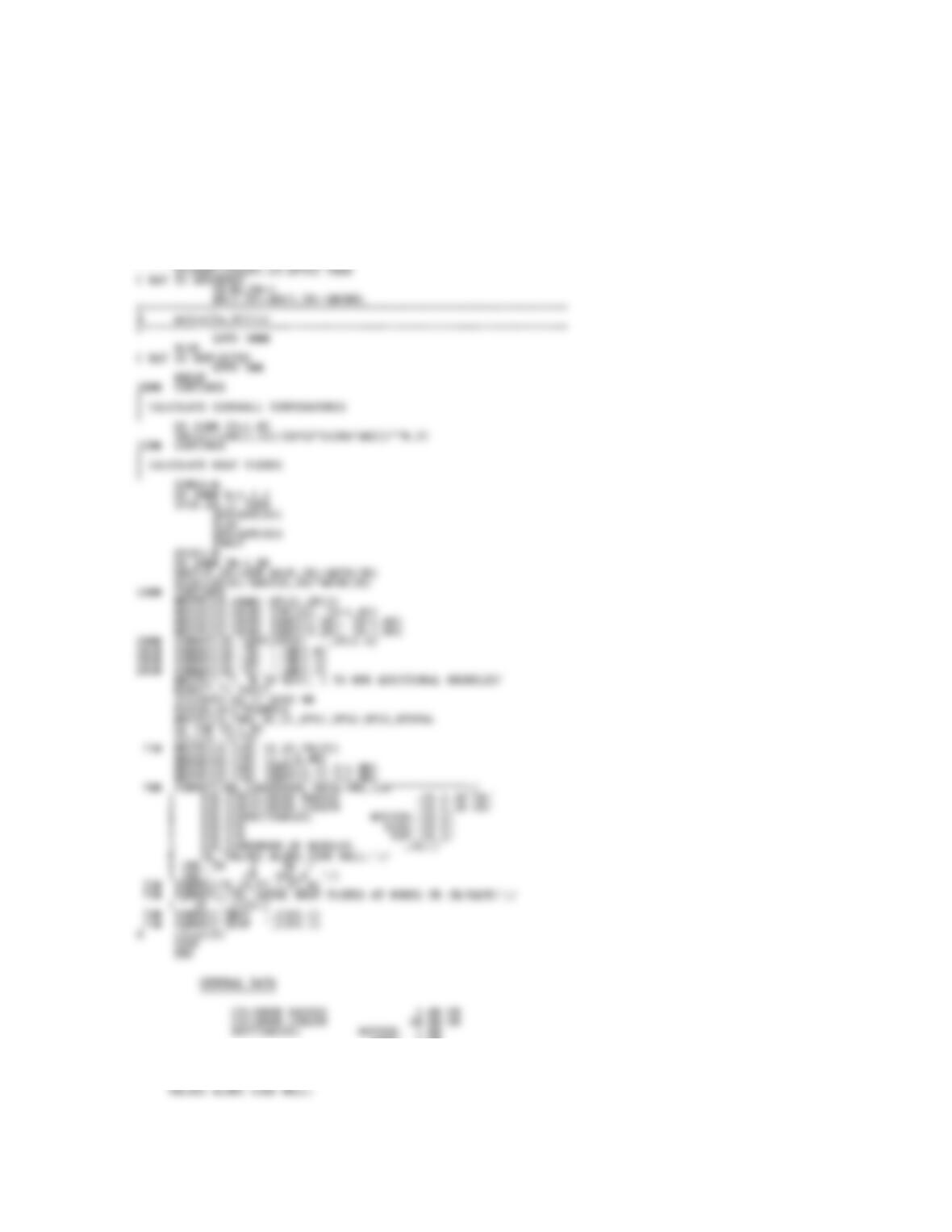CHAPTER 8 263
C*******************************************************************
GOTO 1000
ELSE
C RAY IS REFLECTED
GOTO 100
ENDIF
C
C CHECK FOR POINT OF INTERSECTION WITH SURFACE 3
C600 D=(CL-ZE)/SZ
RL=SQRT((XE+D*SX)**2+(D*SY)**2)
C*******************************************************************
d write(lu,909)rl,d
d909 format(6X,’BUNDLE HITS TOP’,’ RL=’,F5.1,’ D=’,F4.1)
C*******************************************************************
C IS RAY ABSORBED OR REFLECTED?
SIDE: 1.00
TOP: 0.50
NUMBER OF BUNDLES 100617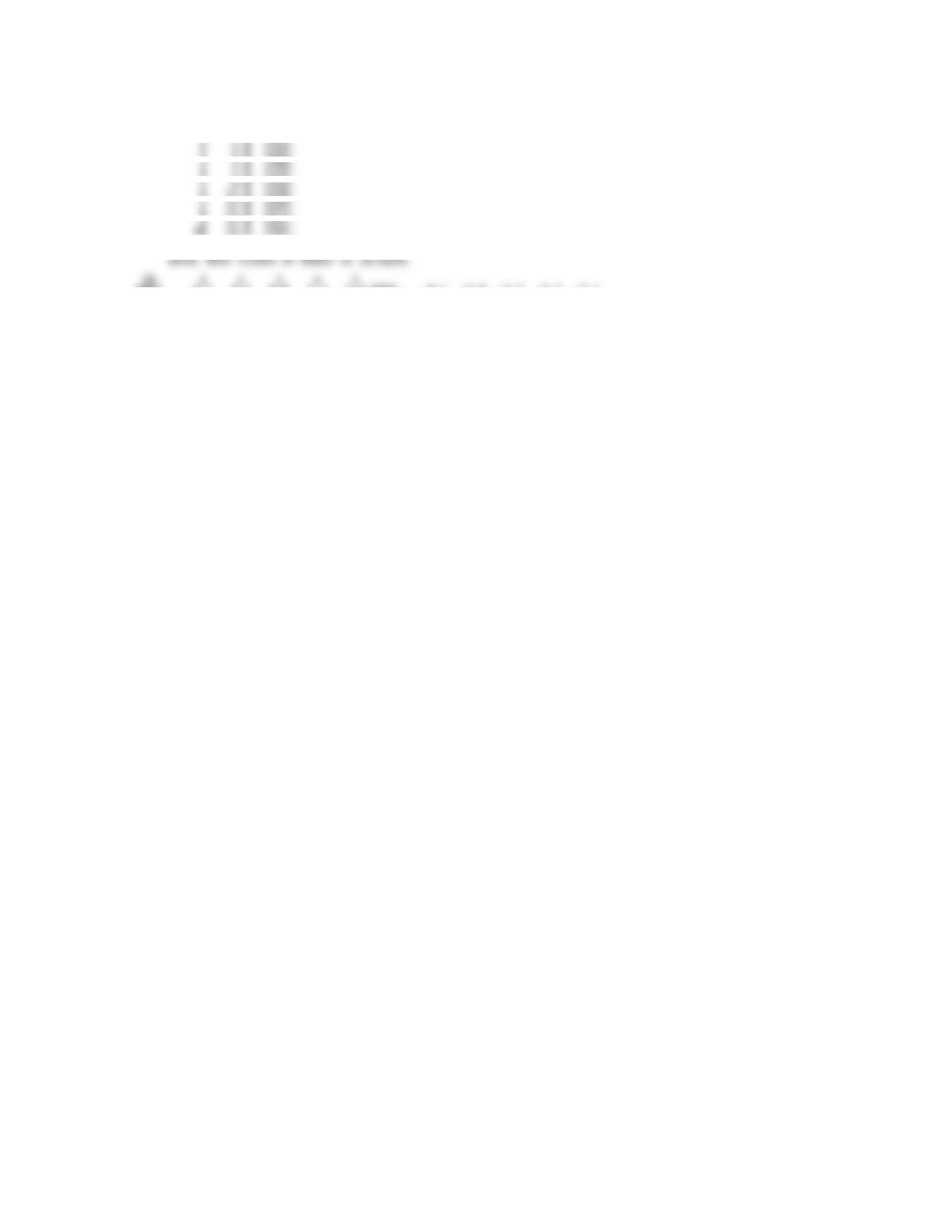IR Z TW
CM DEG.K
2 3.0 1422.
4 7.0 1379.
6 11.0 1328.
8 15.0 1273.
10 19.0 1202.
QBOT 8.5 8.2 8.0 7.3 6.9 QTOP -8.1 -7.8 -7.7 -7.5 -7.1

## Trusted by Thousands ofStudents

Here are what students say about us.